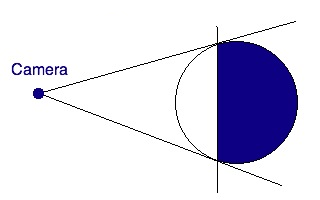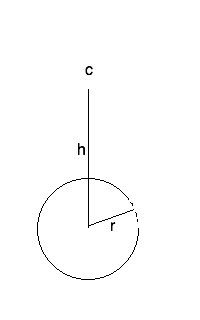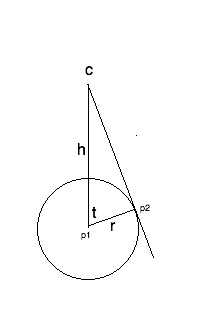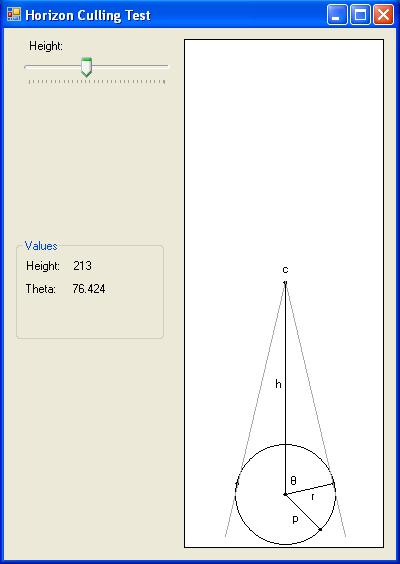# Horizon Culling 2 – The Math

In my previous post on horizon culling we talked about what horizon culling is, the reasons one might want to do it, and went over a simple C# vector class. In this post we’ll take a look at the math we’ll need to use.

In my previous post on horizon culling we talked about what horizon culling is, the reasons one might want to use it, and went over a simple C# vector class. In this post we’ll take a look at the math we’ll need to use.

Referring back to one of our examples from the previous post, it seems like we’d need to calculate the two points where the planet starts curving away – i.e. where the dark blue starts.That’s the initial approach that I took while figuring this out. Â But it turns out there is a much simpler solution that uses some basic information that we have available to us.In this image, c is the camera position, h is the height of the camera above the center of the planet, and r is the planet radius. These are all things we have readily available. Now let’s make a couple of adjustments…If we draw a line segment from the camera to a point tangent to the planet surface, then draw a line from the center to that point, we get a nice pretty right triangle. That makes the angle t look very interesting. That angle represents the point on the horizon where it starts to curve away from what the camera can see. When drawing the planet we can calculate a similar angle for each triangle we’re drawing. Â If that angle is less than t then the triangle is visible to the camera. If it’s greater than t then it’s over the horizon. So, let’s figure out how to calculate that angle.

Dredging through some ancient high school memories we meet up with our old friend Sohcahtoa. I don’t recall the entire story I was taught about Sohcahtoa, but it had something to do with a Native-American (we called them Indians back then) who was gifted at math. It seems there are quite a few different versions of the story, but all you really need to remember is the mnemonic.

We can divide the mnemonic into three sections: soh-cah-toa. The first letter in each section represents a trigonomic function: sin, cosine, and tangent. The next two letters represent the ratio of the two sides of a right triangle that gives you the sin, cosine, and tangent. The letter o stands for the Opposite side, which means the side opposite the angle we’re considering; a is Adjacent, meaning the side adjacent to the angle; h is Hypotenuse, or the longest side. Sohcahtoa expands out to:

Sin = opposite / hypotenuse

For our planet, the side opposite the angle t is the line segment that runs from C to p2. We don’t know the length of that, so it’s pretty much worthless to us. However, we do know the length of the hypotenuse – it’s the height of the camera from the center. We also know the length of the adjacent side – it’s the planet radius. The only one of our trig functions that utilizes the adjacent side and the hypotenuse is Cosine. The cosine of the angle t is the length of the adjacent side divided by the length of the hypotenuse, or cos(t) = r / h.

What we really want though is the actual angle t, not the cosine. For that we have the handy function called arccosine, which returns the angle given a cosine. So our final formula becomes t = arccos(r / h). In C# this becomes:

float t = Math.Acos(r / h);

And since my brain works in degrees instead of radians, we’ll do that conversion as well:

float t = Math.Acos(r / h) * (180.0f / Math.PI);

One thing that’s interesting is that we don’t ever have to find the point p2. The relationship between radius and height only works for right triangles, and the radius never changes, so if we do the divide we’re always going to get the cosine as if we were working with a right triangle. You’ll be able to visualize this a bit better with the final app since you can see p2 moving as you change the height.

And that’s pretty much it. Next time I’ll post the app that lets us visualize this process and I’ll go over how we can use this angle to skip drawing parts of our planet. For now, here’s a screenshot of the app:Not terribly pretty, but it does the job.

## 2 thoughts on “Horizon Culling 2 – The Math”

1.Michelle Ufford says:

Woah! Sohcahtoa! Awesome, I haven’t heard that reference in a long time. 🙂

Another informative and well-written post… thanks, Dave!

2.Michael Swart says:

This reminds me of a math problem that IBM posed a long time ago (It’s the first problem they posed as part of their *Ponder This* monthly math/computer puzzle)

http://domino.research.ibm.com/Comm/wwwr_ponder.nsf/Challenges/May1998.html

Warning, If you’re a certain type of person, you can get addicted to these types of math puzzles.